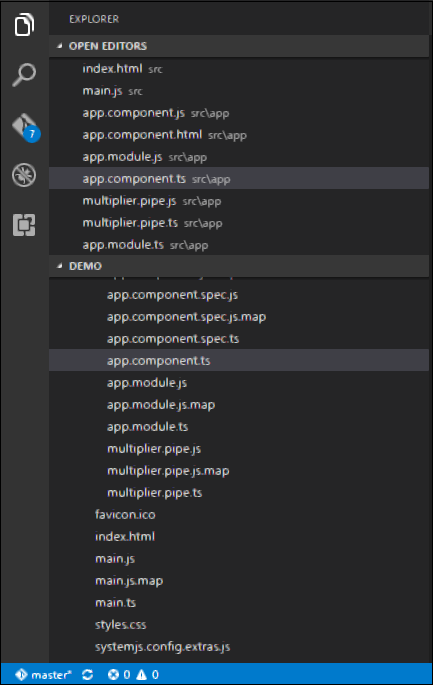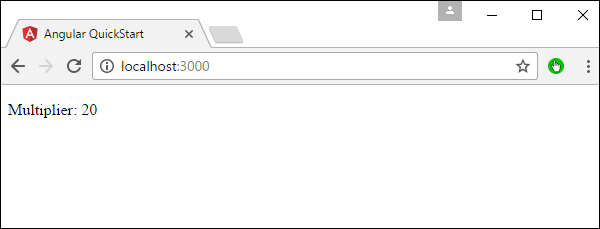# Angular 2 - Custom Pipes

Angular 2 also has the facility to create custom pipes. The general way to define a custom pipe is as follows.

```import { Pipe, PipeTransform } from '@angular/core';
@Pipe({name: 'Pipename'})

export class Pipeclass implements PipeTransform {
transform(parameters): returntype { }
}
```

Where,

• 'Pipename' − This is the name of the pipe.

• Pipeclass − This is name of the class assigned to the custom pipe.

• Transform − This is the function to work with the pipe.

• Parameters − This are the parameters which are passed to the pipe.

• Returntype − This is the return type of the pipe.

Let’s create a custom pipe that multiplies 2 numbers. We will then use that pipe in our component class.

Step 1 − First, create a file called multiplier.pipe.ts.Step 2 − Place the following code in the above created file.

```import {
Pipe,
PipeTransform
} from '@angular/core';

@Pipe ({
name: 'Multiplier'
})

export class MultiplierPipe implements PipeTransform {
transform(value: number, multiply: string): number {
let mul = parseFloat(multiply);
return mul * value
}
}
```

Following points need to be noted about the above code.

• We are first importing the Pipe and PipeTransform modules.

• Then, we are creating a Pipe with the name 'Multiplier'.

• Creating a class called MultiplierPipe that implements the PipeTransform module.

• The transform function will then take in the value and multiple parameter and output the multiplication of both numbers.

Step 3 − In the app.component.ts file, place the following code.

```import {
Component
} from '@angular/core';

@Component ({
selector: 'my-app',
template: '<p>Multiplier: {{2 | Multiplier: 10}}</p>'
})
export class AppComponent {  }
```

Note − In our template, we use our new custom pipe.

Step 4 − Ensure the following code is placed in the app.module.ts file.

```import {
NgModule
} from '@angular/core';

import {
BrowserModule
} from '@angular/platform-browser';

import {
AppComponent
} from './app.component';

import {
MultiplierPipe
} from './multiplier.pipe'

@NgModule ({
imports: [BrowserModule],
declarations: [AppComponent, MultiplierPipe],
bootstrap: [AppComponent]
})

export class AppModule {}
```

Following things need to be noted about the above code.

• We need to ensure to include our MultiplierPipe module.

• We also need to ensure it is included in the declarations section.

Once you save all the code changes and refresh the browser, you will get the following output.